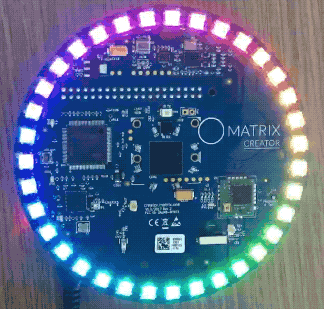# Python Setup

## Prerequisite MATRIX HAL

Make sure you have installed MATRIX HAL, before continuing.

## Python Setup

Install the PIP3 package manager.

```sudo apt-get install python3-pip
```

```python3 -m pip install --upgrade pip
```

Create a project folder.

```mkdir lite_py
cd lite_py
touch app.py
```

Download the matrix-lite-py package. Note that the module to import is called `matrix_lite`.

```sudo python3 -m pip install matrix-lite
```

## Creating An Application

Copy our Hello World example below into `app.py` to test your installation.

```from matrix_lite import led
from time import sleep
from math import pi, sin

everloop = ['black'] * led.length

if len(everloop) == 35:
ledAdjust = 0.51 # MATRIX Creator
else:
ledAdjust = 1.01 # MATRIX Voice

frequency = 0.375
counter = 0.0
tick = len(everloop) - 1

while True:
# Create rainbow
for i in range(len(everloop)):
r = round(max(0, (sin(frequency*counter+(pi/180*240))*155+100)/10))
g = round(max(0, (sin(frequency*counter+(pi/180*120))*155+100)/10))
b = round(max(0, (sin(frequency*counter)*155+100)/10))

everloop[i] = {'r':r, 'g':g, 'b':b}

# Slowly show rainbow
if tick != 0:
for i in reversed(range(tick)):
everloop[i] = {}
tick -= 1

led.set(everloop)

sleep(.035)
```

### Running app.py

Once you have `app.py` ready, use the following command to run our rainbow Hello World.

```python3 app.py
```

### Result## Next Steps

With your device now setup, you can visit our Reference page to get started with MATRIX Lite.